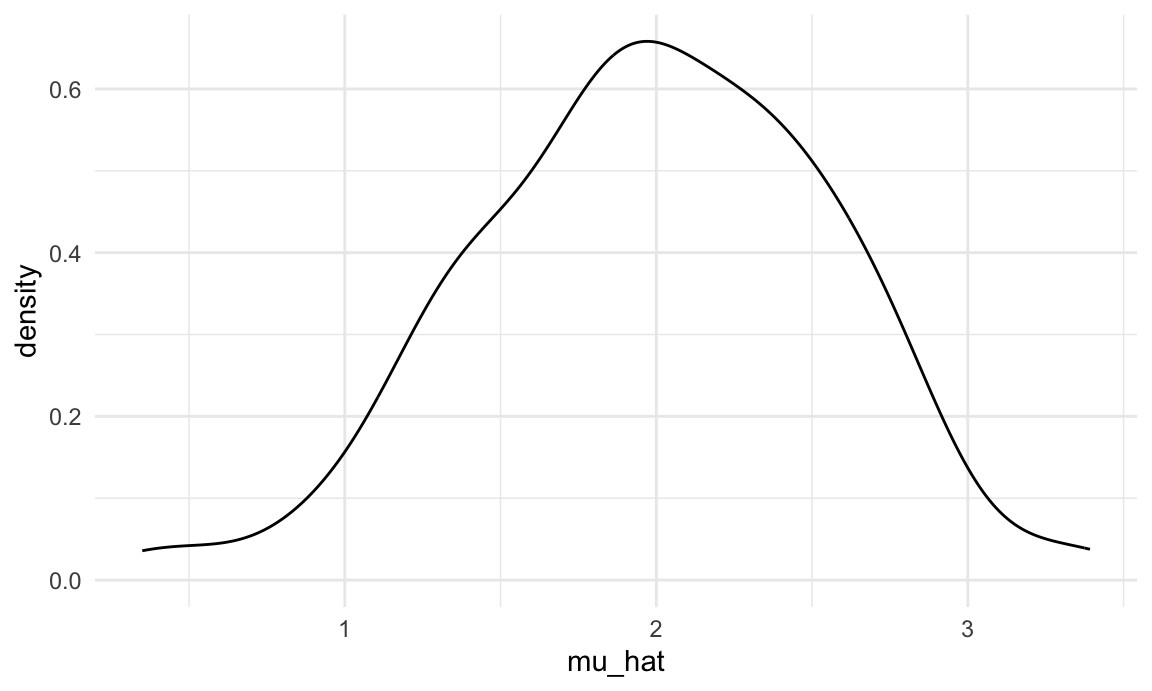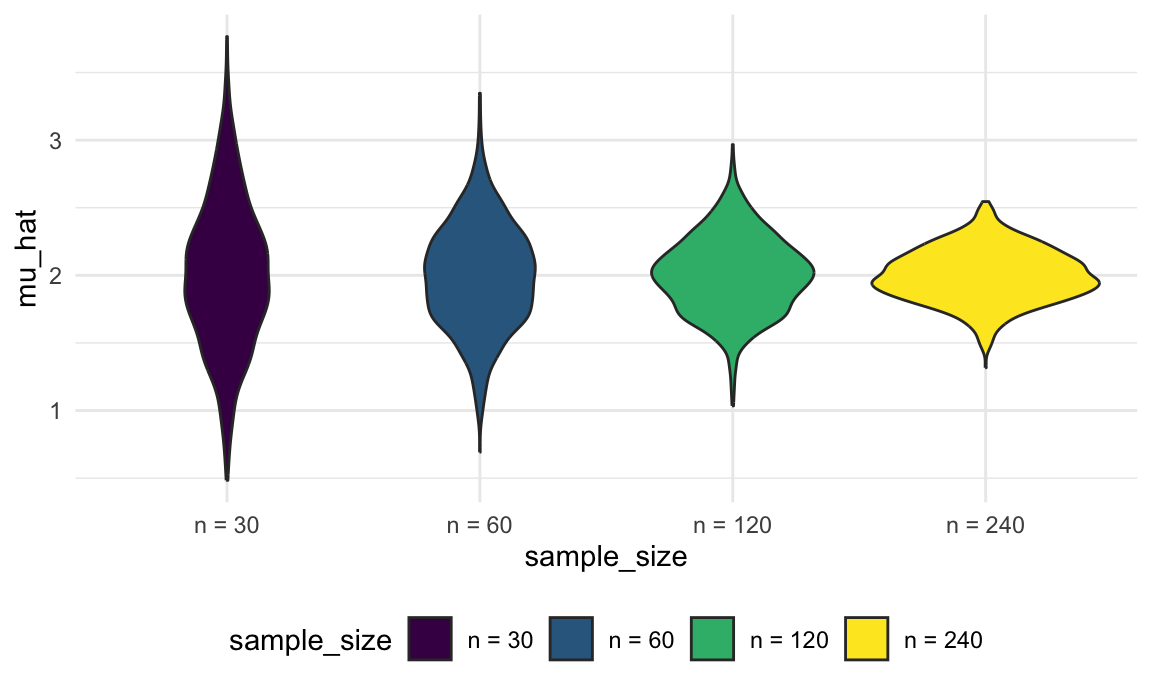We’ve noted that functions are helpful when you repeat code more than twice; we’ve also noted that a lot of statistical methods involve doing the same thing a large number of times. Simulation is a common statistical approach that takes advantage of the ability to iterate many times using computers.

This is the third module in the Iteration topic.

Overview

Learning Objectives

Use iteration methods to simulate data, and explore statistical properties of common estimation methods under repeated sampling using simulations.

Example

I’ll write code for today’s content in a new R Markdown document called simulation.Rmd in the iteration directory / repo. The code chunk below loads the usual packages and sets a seed for reproducibility.

library(tidyverse)

set.seed(1)

Simulation: Mean and SD for one $$n$$

In writing functions we wrote a short function to simulate data from a normal distribution, and return estimates of the mean and standard deviation. Specifically, we generate data from $x_i \sim N[\mu, \sigma]$

for subjects $$1 \leq i \leq n$$ and return estimates

$$\hat{\mu}, \hat{\sigma}$$. That function is below.

sim_mean_sd = function(n, mu = 2, sigma = 3) {

sim_data = tibble(
x = rnorm(n, mean = mu, sd = sigma),
)

sim_data %>%
summarize(
mu_hat = mean(x),
sigma_hat = sd(x)
)
}

Important statistical properties of estimates $$\hat{\mu}$$ are established under the conceptual framework of repeated sampling. If you could draw from a population over and over, your estimates will have a known distribution:

$\hat{\mu} \sim \left[\mu,\frac{\sigma}{\sqrt{n}} \right]$

Because our simulation design generates observations from a Normal distribution we also know that the estimates follow a Normal distribution, although that’s not guaranteed in general. You can do some work to understand the distribution of $$\hat{\sigma}$$, but it’s … messier.

In the real world, drawing samples is time consuming and costly, so “repeated sampling” remains conceptual. On a computer, though, drawing samples is pretty easy. That makes simulation an appealing way to examine the statistical properties of your estimators.

Let’s run sim_mean_sd() 100 times to see the effect of randomness in $$x_i$$ on estimates $$\hat{\mu}, \hat{\sigma}$$.

output = vector("list", 100)

for (i in 1:100) {
output[[i]] = sim_mean_sd(30)
}

sim_results = bind_rows(output)

Taking a look at the for loop we used to create these results, you might notice that there’s no input list – the sequence is used to keep track of the output but doesn’t affect the computation performed inside the for loop. In cases like these, the purrr::rerun function is very handy.

sim_results =
rerun(100, sim_mean_sd(30, 2, 3)) %>%
bind_rows()

Structurally, rerun is a lot like map – the first argument defines the amount of iteration and the second argument is the function to use in each iteration step. As with map, we’ve replaced a for loop with a segment of code that makes our purpose much more transparent but both approaches give the same results.

Let’s make some quick plots and compute some summaries for our simulation results.

sim_results %>%
ggplot(aes(x = mu_hat)) +
geom_density()sim_results %>%
pivot_longer(
mu_hat:sigma_hat,
names_to = "parameter",
values_to = "estimate") %>%
group_by(parameter) %>%
summarize(
emp_mean = mean(estimate),
emp_sd = sd(estimate)) %>%
knitr::kable(digits = 3)
## summarise() ungrouping output (override with .groups argument)
parameter emp_mean emp_sd
mu_hat 1.985 0.567
sigma_hat 2.979 0.384

This is great! We’ve seen how our estimates are distributed under our simulation scenario, and can compare empirical results to theoretical ones. In this way, we can build intution for fundamental statistical procedures under repeated sampling in a way that’s not possible with single data sets.

Simulation: Mean for several $$n$$s

Sample size makes a huge difference on the variance of estimates in SLR (and pretty much every statistical method). Let’s try to clarify that effect through simulating at a few sample sizes.

I’ll start this process with a for loop around the code I established above using rerun (I could start from scratch by nesting one for loop in another for loop, but let’s not).

n_list =
list(
"n_30"  = 30,
"n_60"  = 60,
"n_120" = 120,
"n_240" = 240)

output = vector("list", length = 4)

for (i in 1:4) {
output[[i]] = rerun(100, sim_mean_sd(n_list[[i]])) %>%
bind_rows
}

After this loop, output is a list of 4 data frames; each data frame contains the results of 100 simulations at different sample sizes.

Before we spend time looking at the results of the simulation, let’s recast this using list columns and map. I’ll set up a tibble with the sample sizes I want to investigate, and then use rerun to perform the complete simulation for each sample size. Remember that rerun produces a list, so I’m going to use bind_rows to produce a tibble of simulation results for each sample size. Finally, I’ll unnest the tibbles to produce a standard data frame with no list columns.

sim_results =
tibble(sample_size = c(30, 60, 120, 240)) %>%
mutate(
output_lists = map(.x = sample_size, ~rerun(1000, sim_mean_sd(n = .x))),
estimate_dfs = map(output_lists, bind_rows)) %>%
select(-output_lists) %>%
unnest(estimate_dfs)

Let’s take a look at what we’ve accomplished in our simulations! First I’ll take a look at the distribution of mean estimates across sample sizes.

sim_results %>%
mutate(
sample_size = str_c("n = ", sample_size),
sample_size = fct_inorder(sample_size)) %>%
ggplot(aes(x = sample_size, y = mu_hat, fill = sample_size)) +
geom_violin()These estimates are centered around the truth (2) for each sample size, and the width of the distribution shrinks as sample size grows.

Lastly I’ll look at the empirical mean and variance of these estimates.

sim_results %>%
pivot_longer(
mu_hat:sigma_hat,
names_to = "parameter",
values_to = "estimate") %>%
group_by(parameter, sample_size) %>%
summarize(
emp_mean = mean(estimate),
emp_var = var(estimate)) %>%
knitr::kable(digits = 3)
## summarise() regrouping output by 'parameter' (override with .groups argument)
parameter sample_size emp_mean emp_var
mu_hat 30 1.996 0.291
mu_hat 60 2.011 0.146
mu_hat 120 1.990 0.077
mu_hat 240 2.001 0.038
sigma_hat 30 2.972 0.144
sigma_hat 60 2.998 0.075
sigma_hat 120 2.990 0.037
sigma_hat 240 2.993 0.017

These values are consistent with the formula presented for the distribution of the sample mean. This kind of check is a useful way to support derivations (although they don’t serve as a formal proof in any way).

Other materials

• Problem of small power leading to over estimation has been discussed here among other places

The code that I produced working examples in lecture is here.